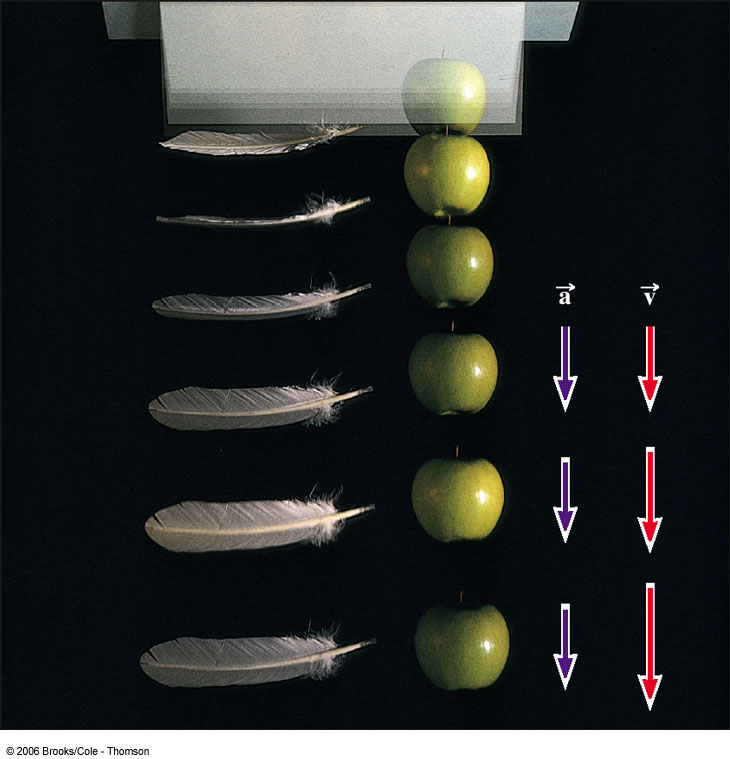# PHYS 170

Lecture 02b - Motion in One Dimension (The Equations of Motion)

## Introduction

Four equations for motion under constant acceleration:

Linear Equations Missing
$v = v_0 + at$ $s$
$s - s_0 = v_0t + \frac{1}{2} a t^2$ $v$
$s - s_0 = \frac{1}{2} (v_0+v)t$ $a$
$v^2 = v_0^2 + 2a(s-s_0)$ $t$

There are six variables overall: $s_0, v_0$ (intial variables), and $s, v, a, t$ (final variables).
Each of the equations is labeled on the right by the final variables that is missing. For example, the first equation has the final variables $v, a, t$ but is missing $s$, so we will call it the $s$-equation.

The meaning of the variables:

Symbol Meaning
$s_0$ initial displacement
$v_0$ initial velocity
$s$ final displacement
$v$ final velocity
$a$ acceleration (constant)
$t$ time

If the acceleration is not a constant (i.e. it changes over time) then the equations are invalid. However, in this course, you can assume $a$ is constant unless the question stated otherwise.

## Acceleration due to Gravity

Gravity pulls everything downward in such a way that the vertical acceleration of an object falling on the surface of the Earth is given by: $$a = -9.8m/s^2$$ The negative sign denotes the fact that gravity always ponts downward. The value of $a$ does not depend on the mass.

The magnitude of the acceleration due to gravity is defined to be $g$: $$g = |a| = 9.8m/s^2$$ Note that $g$ is by definition positive (because it is the magnitude), so never write something like $g=-9.8m/s^2$. Be aware of the difference between the notations of $a$ and $g$, the former is negative and the latter is negative:
• $a = - g= -9.8m/s^2$
• $g=|a|=9.8m/s^2$
• $a = 9.8m/s^2$
• $g=-9.8m/s^2$
Below is a picture of an apple and a piece of feather under free fall inside a vacuum chamber (to eliminate the air resistance). Even though the feather is much lighter than the apple, they both reach the ground at exactly the same time because their downward acceleration is exactly the same.